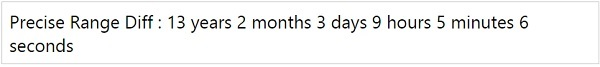# MomentJS - Plugins

Plugins are extended features added on MomentJS. MomentJS is an open source project and many plugins are found in MomentJS which are contributed by its users and available using Node.js and GitHub.

This chapter discusses some of the calendars plugins and date formats plugins available in MomentJS.

## Calendar Plugins

This section discusses two types of Calendar plugins: ISO calendar and Taiwan calendar.

### ISO calendar

You can use the following command to install it with Node.js −

```npm install moment-isocalendar
```

You can get the moment-isocalendar.js from GitHub − https://github.com/fusionbox/moment-isocalendar Observe the following working example with isocalendar and MomentJS −

Example

```var m = moment().isocalendar();
```

Output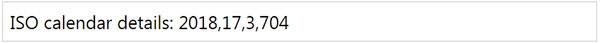Example

```var m = moment.fromIsocalendar([2018, 51, 10, 670]).format('LLLL');
```

Output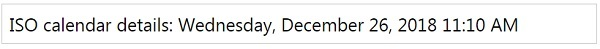### Taiwan Calendar

You can use the following command to install it with Node.js −

```npm install moment-jalaali
```

You can get the moment-taiwan.js from GitHub − https://github.com/bradwoo8621/moment-taiwan Observe the following working example with isocalendar and MomentJS −

Example

```var m = moment('190/01/01', 'tYY/MM/DD');
var c = m.twYear();
```

Output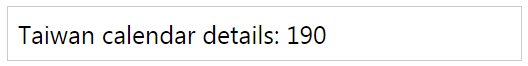## Date formats Plugins

This section discusses the following types of Date format plugins −

• Java dateformat parser
• Short date formatter
• Parse date format
• Duration format
• Date Range
• Precise Range

### Java DateFormat Parser

You can use the following command to install it with Node.js −

You can get the moment-jdateformatparser.js from GitHub − https://github.com/MadMG/moment-jdateformatparser Observe the following working example for moment-jdateformatparser and MomentJS −

Example

```var m = moment().formatWithJDF("dd.MM.yyyy");
```

Output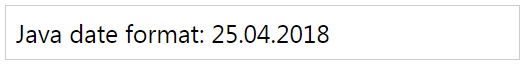### Short date formatter

The JavaScript file for shortdateformat can be fetched from GitHub −

https://github.com/researchgate/moment-shortformat

Syntax

```moment().short();
```

The display looks like as shown in the table here −

From moment From moment().short()
0 to 59 seconds 0 to 59 s
1 to 59 minutes 1 to 59 m
1 to 23 hours 1h to 23h
1 to 6 days 1d to 6d
>= 7 days and same year Display will be like such as feb 3, mar 6
>= 7 days and diff year Display will be like such as feb 3, 2018, mar 6, 2018

You can take the script for momentshort from GitHub link given above.

Example

```var a = moment().subtract(8, 'hours').short();
var b = moment().add(1, 'hour').short(true);
```

Output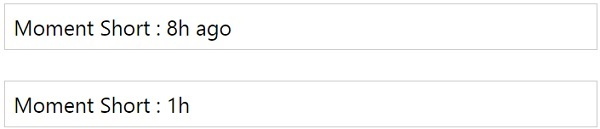If you want to remove the suffix ago or in, you can pass true to short(tru.

### Parse date format

You can use the following command to install it with Node.js −

```npm install moment-parseformat
```

Example

```var a = moment.parseFormat('Friday 2018 27 april 10:28:10');
```

Output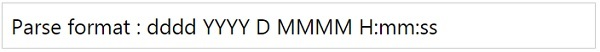Observe that the output shows that whatever parameters (date/ time) is given to the parseFormat, it gives the format of the date as shown above.

### Duration Format

You can use the following command to install duration format on Node.js −

The repository for duration format is available here − https://github.com/jsmreese/moment-duration-format Let us see a working example with duration format −

Example

```var a = moment.duration(969, "minutes").format("h:mm:ss");
```

Output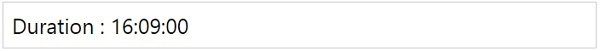This adds more details to the duration on moment created.

### Date Range

You can use the following command to install date range on Node.js −

```npm install moment-range
```

Example

```window['moment-range'].extendMoment(moment);
var start = new Date(2012, 0, 15);
var end = new Date(2012, 4, 23);
var range = moment.range(start, end);
console.log(range.start._d);
console.log(range.end._d);
```

Output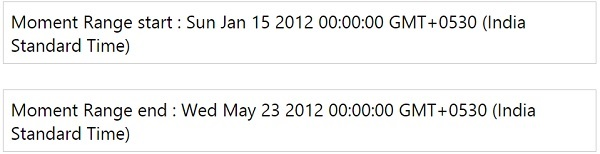### Precise Range

Precise range will display the exact date difference in date, time and in human readable format. You can use the following command to install precise range on Node.js −

```npm install moment-precise-range-plugin
```

Example

```var a = moment("1998-01-01 09:00:00").preciseDiff("2011-03-04 18:05:06");
```

Output